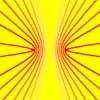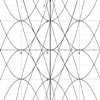# Resources tagged with: Quadratic functions and graphs

Filter by: Content type:
Age range:
Challenge level:

### There are 14 results

Broad Topics > Coordinates, Functions and Graphs > Quadratic functions and graphs### Parabolic Patterns

##### Age 14 to 18 Challenge Level:

The illustration shows the graphs of fifteen functions. Two of them have equations y=x^2 and y=-(x-4)^2. Find the equations of all the other graphs.### More Parabolic Patterns

##### Age 14 to 18 Challenge Level:

The illustration shows the graphs of twelve functions. Three of them have equations y=x^2, x=y^2 and x=-y^2+2. Find the equations of all the other graphs.##### Age 16 to 18 Challenge Level:

In y = ax +b when are a, -b/a, b in arithmetic progression. The polynomial y = ax^2 + bx + c has roots r1 and r2. Can a, r1, b, r2 and c be in arithmetic progression?### Parabolas Again

##### Age 14 to 18 Challenge Level:

Here is a pattern composed of the graphs of 14 parabolas. Can you find their equations?### Grid Points on Hyperbolas

##### Age 16 to 18 Challenge Level:

Find a condition which determines whether the hyperbola y^2 - x^2 = k contains any points with integer coordinates.### Parabella

##### Age 16 to 18 Challenge Level:

This is a beautiful result involving a parabola and parallels.##### Age 14 to 18 Challenge Level:

This task develops knowledge of transformation of graphs. By framing and asking questions a member of the team has to find out which mathematical function they have chosen.### ' Tis Whole

##### Age 14 to 18 Challenge Level:

Take a few whole numbers away from a triangle number. If you know the mean of the remaining numbers can you find the triangle number and which numbers were removed?### Guessing the Graph

##### Age 14 to 16 Challenge Level:

Can you suggest a curve to fit some experimental data? Can you work out where the data might have come from?### Minus One Two Three

##### Age 14 to 16 Challenge Level:

Substitute -1, -2 or -3, into an algebraic expression and you'll get three results. Is it possible to tell in advance which of those three will be the largest ?### Consecutive Squares

##### Age 14 to 16 Challenge Level:

The squares of any 8 consecutive numbers can be arranged into two sets of four numbers with the same sum. True of false?### Integral Inequality

##### Age 16 to 18 Challenge Level:

An inequality involving integrals of squares of functions.### Converse

##### Age 14 to 16 Challenge Level:

Clearly if a, b and c are the lengths of the sides of an equilateral triangle then a^2 + b^2 + c^2 = ab + bc + ca. Is the converse true?### Geometric Parabola

##### Age 14 to 16 Challenge Level:

Explore what happens when you draw graphs of quadratic equations with coefficients based on a geometric sequence.